Latest news 2023-05-22: new blog post "Bug Bounty Hunters".

## 9.4.5 Fractions

Fractions are created using the command

\frac{<numerator>}{<denominator>}

The amsmath package also provides the command

\cfrac[<pos>]{<numerator>}{<denominator>}

which is designed for continued fractions. The optional argument pos can be used for left (l) or right (r) placement of any of the numerators. (The default is centred.)

Example:

A simple fraction:

Produces: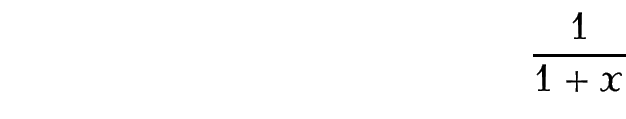Compare with:

In-line: $\frac{1}{1+x}$

which produces: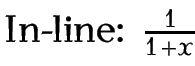Example (Nested):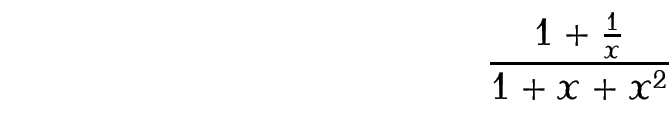Example (Continued Fraction);

A continued fraction (example taken from amsmath documentation and uses \sqrt, described in §9.4.6. Roots, and \dotsb, described in §9.4.7. Mathematical Symbols):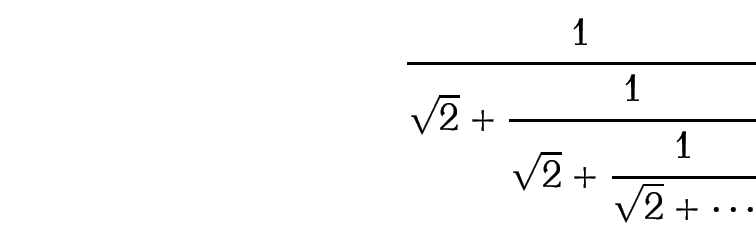Example (A Derivative):

$f'(x) = \frac{df}{dx}$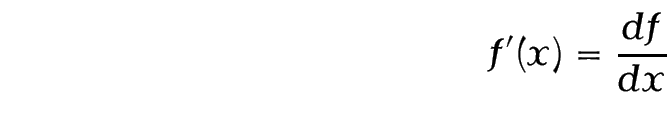As with “e”, the differential operator “d” should be in an upright font as it is not a variable: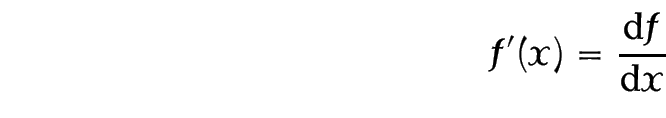The above example is rather cumbersome, particularly if you have a lot of derivatives, so it might be easier to define a new command. In the preamble define:

Then in the document:

$f'(x) = \deriv{f}{x}$Example (Partial Derivative):

Partial derivatives can be obtained similarly using the command \partial to display the partial derivative symbol. As in the previous example, first define a new command to format a partial derivative in the preamble:

\newcommand{\pderiv}{\frac{\partial #1}\partial #2}

Then in the document:

$f_x = \pderiv{f}{x}$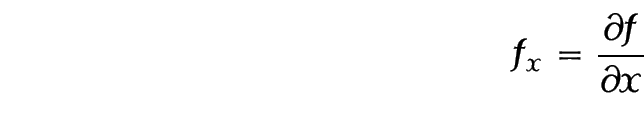Example (Double Partial Derivative):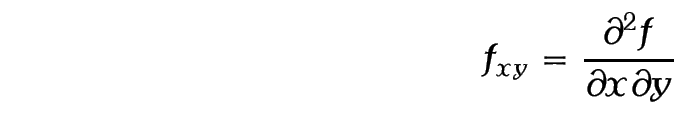Example (First principles):

$f'(x) = \lim_{\Delta x \to 0} \frac{f(x + \Delta x)-f(x)}{\Delta x}$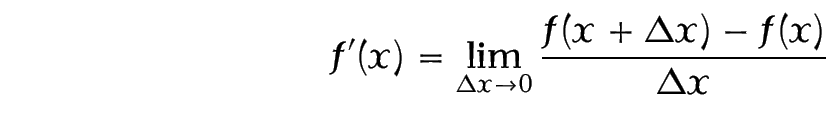This book is also available as A4 PDF or 12.8cm x 9.6cm PDF or paperback (ISBN 978-1-909440-00-5).

© 2012 Dickimaw Books. "Dickimaw", "Dickimaw Books" and the Dickimaw parrot logo are trademarks. The Dickimaw parrot was painted by Magdalene Pritchett.